1.Which pair of compounds could be used in the preparation of calcium sulfate?

2.Which equation describes the most suitable reaction for making lead sulphate?

3.An excess of copper (II) oxide is added to dilute sulfuric acid to make crystals of hydrated copper(II) sulfate.The processes listed may be used to obtain crystals of hydrated copper (II) sulfate.

1. Concentrate the resulting solution
2. Filter
3. Heat the crystals
4. Wash the crystalsWhich processes are needed and in which order?

4. Molten lead (II) bromide is electrolysed as shown.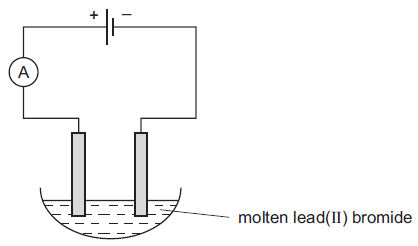Which ions are discharged at each electrode?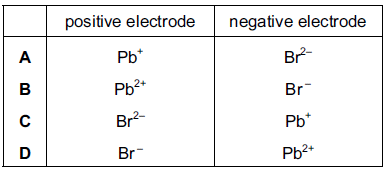5. The following statements about dilute sulphuric acid are all correct.

1 A white precipitate is formed when aqueous barium chloride is added.

2 The solution turns anhydrous copper (II) sulphate from white to blue.

3 Addition of Universal Indicator shows that the solution has a pH value of less than 7.0.

4 The solution reacts with copper (II) oxide, forming a blue solution.

Which two statements confirm the acidic nature of the solution?

6.One mole of a sample of hydrated sodium sulphide contains 162 g of water of crystallisation.What is the correct formula of this compound?

6.The diagram shows the electrolysis of a concentrated aqueous solution containing both Copper (II) ions and sodium ions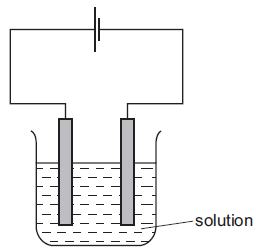Which metal is deposited at the negative electrode and why?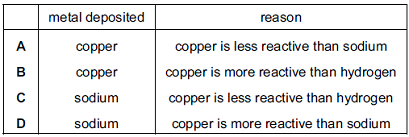8.The diagram shows the apparatus used to electrolyse lead (II) bromide using inert electrodes.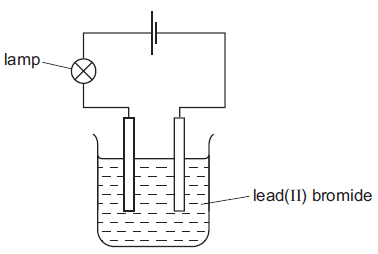Why does the lamp light up only when the lead(II) bromide is melted?

9.Which two substances conduct electricity?

10.A sample of copper contains a metal impurity which is below copper in the reactivity series.The diagram shows the apparatus used for refining the sample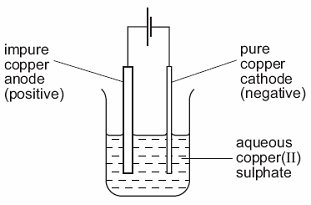The loss in mass of the anode (positive electrode) is 50 g and the gain in mass of the cathode (negative electrode) is 45 g.

What is the percentage purity of this sample of copper?

11.In the extraction of iron, carbon monoxide acts as

12.Which statement describes a test for carbon dioxide gas?

13.Which statement about diamond and graphite is correct?

14.Carbon dioxide is produced when dilute hydrochloric acid reacts with

15.What does not happen in the combustion of pure ethane in a plentiful supply of air?

16.Carbon and chlorine form a chloride.What is the formula of this chloride?

17. Which statement about diamond and graphite is correct?

18.Which of the following does not produce carbon dioxide?

19.Which of the following does not produce carbon dioxide?

20.A solid substance Z burns in air to form a product that is gaseous at 20 °C.What is Z?

Question 1 of 20